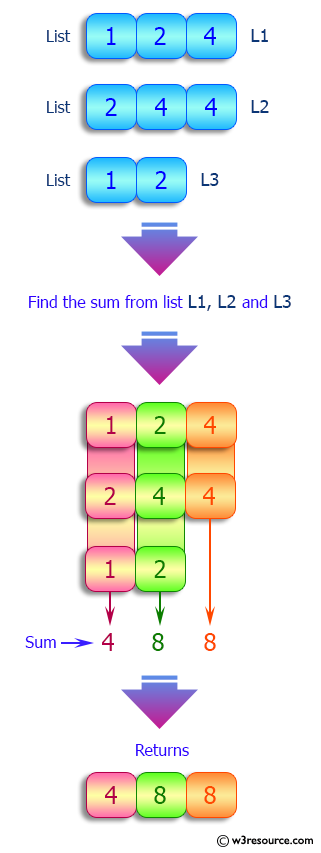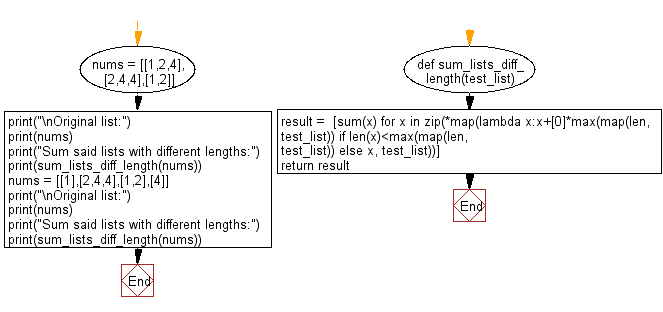﻿ Python: Sum two or more lists, the lengths of the lists may be different - w3resource# Python: Sum two or more lists, the lengths of the lists may be different

## Python List: Exercise - 194 with Solution

Write a Python program to sum two or more lists, the lengths of the lists may be different.

Sample Solution:

Python Code:

``````def sum_lists_diff_length(test_list):
result =  [sum(x) for x in zip(*map(lambda x:x+*max(map(len, test_list)) if len(x)<max(map(len, test_list)) else x, test_list))]
return result

nums = [[1,2,4],[2,4,4],[1,2]]
print("\nOriginal list:")
print(nums)
print("Sum said lists with different lengths:")
print(sum_lists_diff_length(nums))

nums = [,[2,4,4],[1,2],]
print("\nOriginal list:")
print(nums)
print("Sum said lists with different lengths:")
print(sum_lists_diff_length(nums))
```
```

Sample Output:

```Original list:
[[1, 2, 4], [2, 4, 4], [1, 2]]
Sum said lists with different lengths:
[4, 8, 8]

Original list:
[, [2, 4, 4], [1, 2], ]
Sum said lists with different lengths:
[8, 6, 4]
```

Pictorial Presentation:Flowchart:## Visualize Python code execution:

The following tool visualize what the computer is doing step-by-step as it executes the said program:

Python Code Editor:

Have another way to solve this solution? Contribute your code (and comments) through Disqus.

What is the difficulty level of this exercise?

Test your Python skills with w3resource's quiz

﻿

## Python: Tips of the Day

Floor Division:

When we speak of division we normally mean (/) float division operator, this will give a precise result in float format with decimals.

For a rounded integer result there is (//) floor division operator in Python. Floor division will only give integer results that are round numbers.

```print(1000 // 300)
print(1000 / 300)```

Output:

```3
3.3333333333333335```